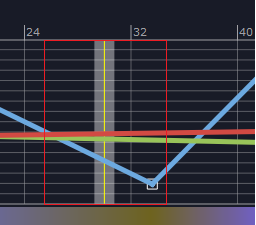QGraphicsView and items, I don’t know how to describe the problem correctly, so I couldn’t find the answer

• hi all, i have a problem
i have a background item on scene, and cursor item whose parent is background item, QGraphicsView resizeEvent calls setTransform of the background item

QTransform matrix(background_item_->transform());
matrix.scale(this->transform().m11() / scale_facor_x_, this->transform().m22() / scale_factor_y_);
QRectF view_rect(mapToScene(this->viewport()->rect()).boundingRect());

scale_facor_x_ = (view_rect.width() / background_item_->boundingRect().width();
scale_factor_y_ = (view_rect.height() / background_item_->boundingRect().height();

matrix.scale(scale_facor_x_, scale_factor_y_);

background_item_->setTransform(matrix);
background_item_->setPos(QPointF(0, 0));

after this cursor position updates, but cursor has flag ItemIgnoresTransformations and fixed width 20

cursor_item_->setRect(QRectF(QPointF(0, 0), QSizeF(20, this->sceneRect().height())));
cursor_item_->setPos(cursor_item_->pos().x(), background_item_->mapFromScene(QPointF(0, 0)).y());

in mousePressEvent I need to check if the cursor is catching, but the cursor rect is wider than the visible rect, so the cursor is grabbed in a larger area like in the screenshotQRectF CursorItem::boundingRect() const
{
return QRectF(this->rect().x() - this->rect().width() / 2, this->rect().y(), this->rect().width(), this->rect().height());
}